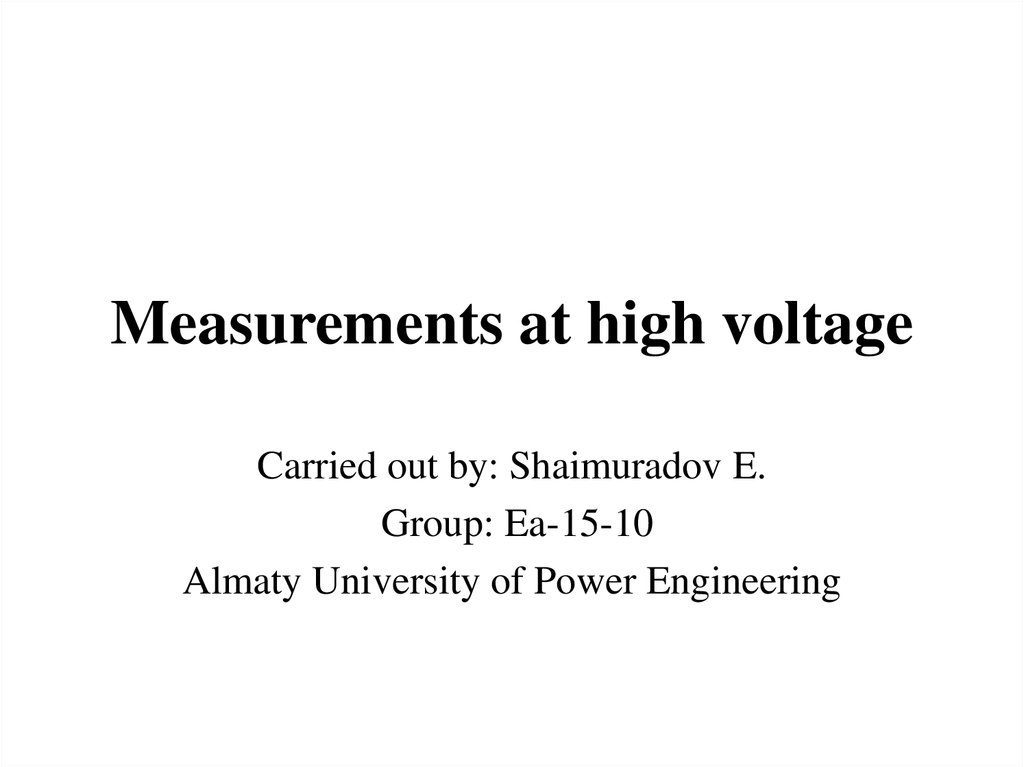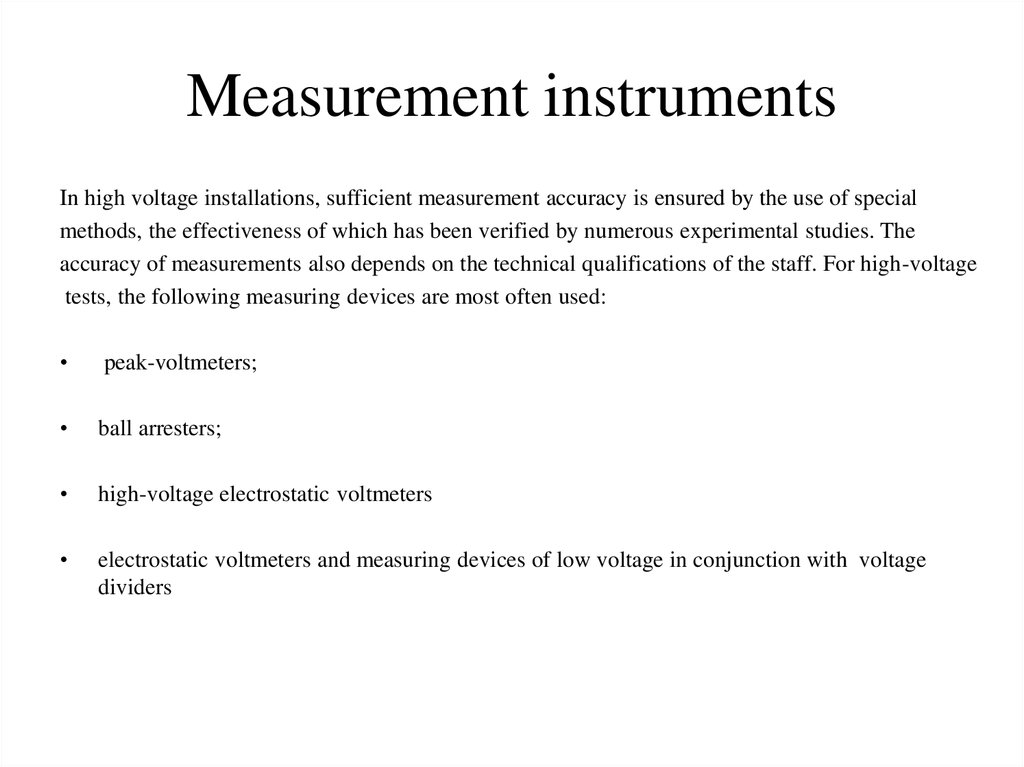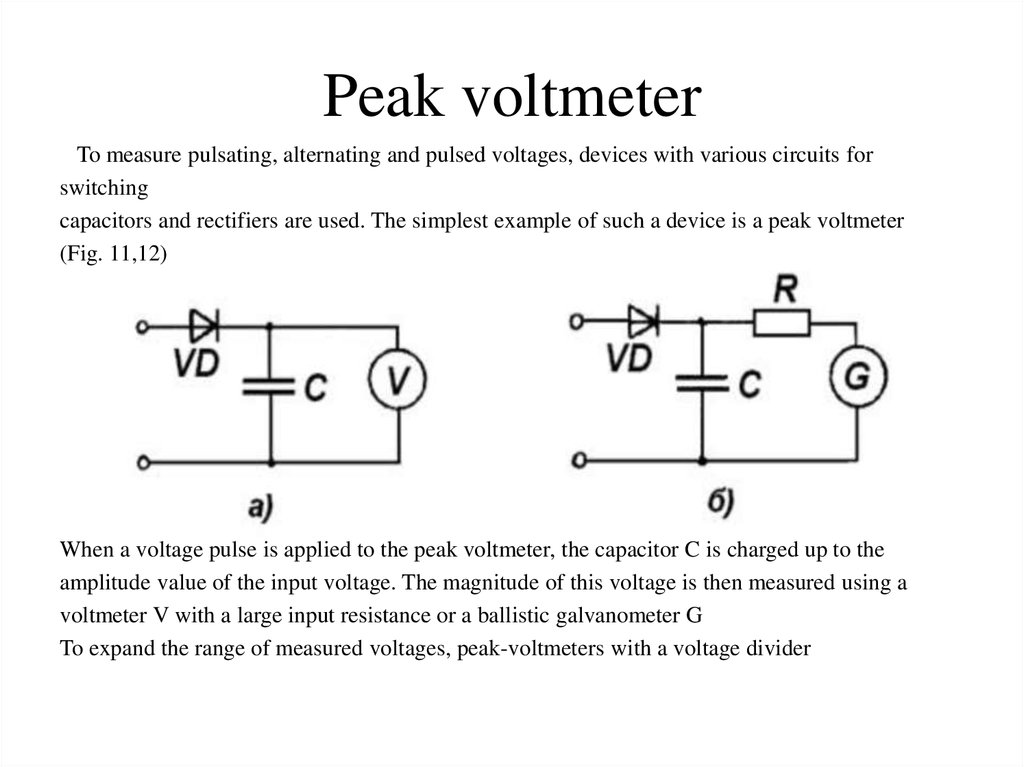# Measurements at high voltage

## 1. Measurements at high voltage

Group: Ea-15-10
Almaty University of Power Engineering

## 2. Measurement instruments

In high voltage installations, sufficient measurement accuracy is ensured by the use of special
methods, the effectiveness of which has been verified by numerous experimental studies. The
accuracy of measurements also depends on the technical qualifications of the staff. For high-voltage
tests, the following measuring devices are most often used:
peak-voltmeters;
ball arresters;
high-voltage electrostatic voltmeters
electrostatic voltmeters and measuring devices of low voltage in conjunction with voltage
dividers

## 3. Peak voltmeter

To measure pulsating, alternating and pulsed voltages, devices with various circuits for
switching
capacitors and rectifiers are used. The simplest example of such a device is a peak voltmeter
(Fig. 11,12)
When a voltage pulse is applied to the peak voltmeter, the capacitor C is charged up to the
amplitude value of the input voltage. The magnitude of this voltage is then measured using a
voltmeter V with a large input resistance or a ballistic galvanometer G
To expand the range of measured voltages, peak-voltmeters with a voltage divider

## 4. Ball arresters

The essence of measuring high voltage with a ball discharger is that a discharge in a weakly
inhomogeneous field between two spherical electrodes in the air arises at a certain voltage with a
small spread and a small delay.
The discharge voltage for a given diameter of the balls and the method of their inclusion
depends linearly on the distance between the balls. A high-voltage amplitude value is measured
using aball-gage.
The discharge voltage depends on pressure, temperature and humidity. In the standard tables,
breakdown voltages Ubr are given, corresponding to a normal pressure of 760 mm Hg. (100 kPa)
and a normal temperature of 20 ° C. If the pressure p and the temperature t differ from the normal
ones, the breakdown voltage Ubrb is determined by the formula:

## 5. For distances d between balls to 0.5d (where D is the diameter of the ball), the table gives the value of the discharge voltage

with an error not exceeding ± 3%, and for distances from 0.5D to
0.75D with a greater error. At distances greater than 0.75D, measurements by balls are not
recommended. Thus, the following inequalities must be satisfied in the measurements:
To protect the surface of the balls from fusion and erosion, as well as to suppress high-frequency
oscillations during breakdowns, an additional resistance of 0.1-0.0 MΩ should be connected in
series with the ball discharger.
The wide application of ball gaps to measure high voltages is explained by the simplicity of
their arrangement and the accuracy acceptable for practice. However, a measurement accuracy of
± 3% is only possible with a strictly spherical surface of the electrodes. The diameter of the balls
should not differ from the standard by more than ± 0.1%. Surfaces of balls should be polished,
dry and clean.
When measuring impulse voltages, one should take into account the influence on the accuracy
of measuring the pulse duration of the acting voltage, the steepness of its front and polarity.

## 6. Electrostatic voltmeters

Electrostatic voltmeters are used to measure the effective values ​of steady-state or
periodically
varying voltages. The principle of operation of electrostatic voltmeters is based on the use of the
interaction force between two electrodes in the electric field of the measured voltage. The
voltmeter S-96 (for voltages up to 30 kV) and S-100 (for voltages up to 75 kV) with an error
of no more than 1.5% are widely used for measurement. Voltage dividers are used to expand the
range of the measured voltages of electrostatic voltmeters and to measure the measured quantitie
s using oscilloscopy. Voltage dividers make it possible to record, with the help of low-voltage
measuring devices (voltmeters, oscilloscopes, etc.) practically unlimited in amplitude constant,
alternating and impulse voltages The part of the divider parallel to which the
measuring device is switched on is considered to be the low-voltage divider arm. The rest is called
the high-voltage divider arm. The ratio of the voltage at the input of the divider U1, to the voltage
on the measuring instrument U2, is called the division coefficient of the divider.
To ensure the fixation of the process under investigation in high-voltage installations without
distortion, voltage dividers must satisfy the following conditions:
• 1) when connected to the circuit under test, do not influence the amplitude and shape of the
measured voltage;
• 2) have a pressure coefficient that does not depend on the frequency and amplitude of the
measured voltage in the working range of variation of these quantities, and also on the change
in external atmospheric conditions

## 7. 3) on the divider elements there should not be a crown, and the leakage currents through the insulation must be much less than

the operating current in the circuit of the divider itself.
Voltage dividers are active, capacitive and combined. To measure a rectified or alternating
voltage of an industrial frequency, active dividers made of wire or non-wire resistors can be
successfully used. For wire resistors use nichrome, constantan, manganin. An obligatory
condition for the manufacture of wire active voltage dividers is bifilar winding of the wire in
order to reduce the inductance of the divider.
To reduce the error in the fission factor caused by leakage through the insulation and the
crown, the resistance of the active divider is adopted so that the current flowing through it is
within 1-10 mA. In those cases where greater measurement accuracy is not required, non-wire
resistors of composite type are used to manufacture active voltage dividers. On an alternating
current, the measurement error in the case of active divisors is sharply increased due to the
influence of parasitic capacitances.
To measure high voltages of the industrial frequency, capacitor dividers are used more often,
which, compared to the active ones, have smaller dimensions and cost. An important
advantage of capacitive dividers is the insignificant effect on their coefficient of fission of
parasitic capacitances and negligible consumption of active energy. In capacitive dividers,
capacitors with ceramic dielectrics with negligible inductance and high electrical strength are
most often used, for example, ceramic capacitors K15U

## 8. All types of dividers can be used to fix pulse voltages, however, when using active divisors, the measurement error due to the

inductance of resistors and parasitic capacitances is usually higher.
To reduce the effect of parasitic capacitance on the dividing ratio of the divider, screen electrodes
are used, which help to reduce the capacitive currents in its circuit. Typically, for this purpose, an
annular electrode is placed slightly below the high-voltage input of the divider and connected to it
by several radial conductors. An active divider with such a screen makes it possible to record a cut
off voltage wave of less than 1 μs with an error of 2-3%.

## 9.

THANKS FOR ATTENTION